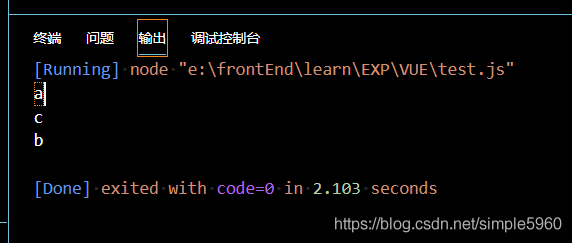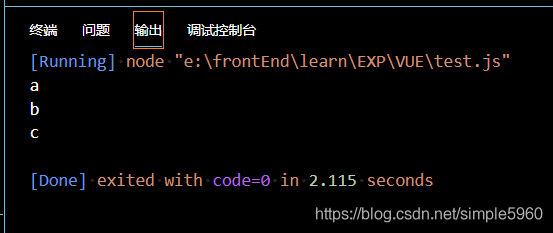# Deep understanding of async await asynchronous programming synchronization

we know that the JavaScript code executes sequentially, so let’s look at the result of the execution of the following code:

``````function a(){
return new Promise((resolve,reject)=>{
resolve("a")
})
}
function b(){
return new Promise((resolve,reject)=>{
setTimeout(() => {
resolve("b")
}, 2000);
})
}
function c(){
return new Promise((resolve,reject)=>{
resolve("c")
})
}
function execute(){
let a1= a()
a1.then(res=>{
console.log(res);
})
let b1= b()
b1.then(res=>{
console.log(res);
})
let c1= c()
c1.then(res=>{
console.log(res);
})
}
execute()
``````so how to show it in order, that is, C wait for B to execute after the execution. The following

``````function a(){
return new Promise((resolve,reject)=>{
resolve("a")
})
}
function b(){
return new Promise((resolve,reject)=>{
setTimeout(() => {
resolve("b")
}, 2000);
})
}
function c(){
return new Promise((resolve,reject)=>{
resolve("c")
})
}
async function execute(){
let a1=await a()
//用了await之后，就不用.then了直接得到结果
console.log(a1)
let b1=await b()
console.log(b1)
let c1=await c()
console.log(c1)
}
execute()
``````